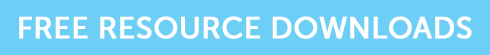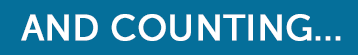Written by Mark Underdown

Bond prices and interest rates are inversely related. This means they move in opposite directions to each other.

When interest rates rise, the price of bonds falls and vice versa.

To help understand how these rate changes affect prices, a concept called ‘duration’ has been developed.

Duration basically measures this relationship and approximates how much bond prices are likely to change when interest rates do. It’s a measure of the bond’s sensitivity to interest rate changes, or you could class it as the bond’s interest rate risk.

The duration measure is in years and usually, the higher the duration of a bond (how far into the future you need to wait for repayment of your principal), the more volatile the bond price will be to changes in interest rates.

### The duration rule of thumb

As a general guide, for each 1% change in interest rates, the bond price will change approximately 1% in the opposite direction for every year of duration.

For example, if a bond has duration of 3 years and interest rates increase by 1%, the bond price will decline approximately 3%.

### Learning the formulas

In our technology-enabled world of large amounts of information, you can conveniently find the quoted figures for duration on financial websites, however it’s good to know the formulas to understand what is going on underneath the bonnet and truly understand the bond/interest rate relationship.

1. ### Macaulay Duration

Frederick Macaulay developed the basic measure of duration in 1938.

Macaulay Duration is the weighted average term to maturity of the cash flows from a bond. The weight of each cash flow is determined by dividing the present value of the cash flow by the price.

Using the text format of learning formulas I highlighted in an earlier article, this is how it’s calculated:### Breaking down an example calculation

A bond pays an annual coupon of 9% and is redeemable at par in 3 years.
Calculate the duration of the bond if interest rates are 8%.Visually, the cash flow of a bond and the duration looks a little like this:1. ### Modified Duration

Whilst Macaulay duration would indicate which bonds are more sensitive to interest rate changes, it wouldn’t quantify how much the prices would move. The next step is to use modified duration.

This calculates the percentage change in the price of a bond for a 1% change in interest rates.  Here’s how it’s calculated:### Applying duration to UK Gilts

Earlier last year, I calculated the approximate interest rate risk applicable to the UK Gilt market. As yields are extremely low, only modest increases in interest rates will significantly impact the attractiveness of gilts in general. Here’s a table from January 2015 that indicates the implied loss of interest rates returned to a modest 4%:Original gilt and modified duration data sourced from www.dmo.gov.uk

### Remember it’s a rule of thumb

Firstly, duration is an approximate measure and tends to over-estimate the fall in a bond’s price, and under-estimate the rise in a bond’s price. This error can be calculated using convexity, which I won’t go into.

It’s also important to understand that interest rates are only one of the factors affecting bond prices, however duration remains key when assessing the risk of your bond portfolio.

### Grab the resources you need!

If you’re studying for your CII R02 exam, and you’re wanting to secure a pass, grab our free taster to try out one of Brand Financial Training’s resources for yourself.  Click the link to download the R02 mock paper taster now!